# Volume Of A Sphere

Here we will learn about the volume of a sphere, including how to calculate the volume of a sphere given its radius and how to find the volume of a hemi-sphere.

There are also volume of a sphere worksheets based on Edexcel, AQA and OCR exam questions, along with further guidance on where to go next if you’re still stuck.

## What is the volume of a sphere?

The volume of a sphere is the amount of space inside a sphere.

To calculate the volume of a sphere, we use the formula,

V=\frac{4}{3} \pi r^3 .

Notice the cube of the radius (r^3) is in the volume formula. Volume is a measure in three-dimensions so the units for the volume are units cubed.

For example, find the volume of the sphere with a radius of 5 \; cm .

\begin{aligned} \text{Volume}&=\frac{4}{3} \pi r^3 \\\\ &= \frac{4}{3} \times \pi \times 5^3\\\\ &=\frac{500}{3}\pi\\\\ &=524.6 \ cm^3 \ \text{to 1 dp} \end{aligned}

### What is the volume of a sphere?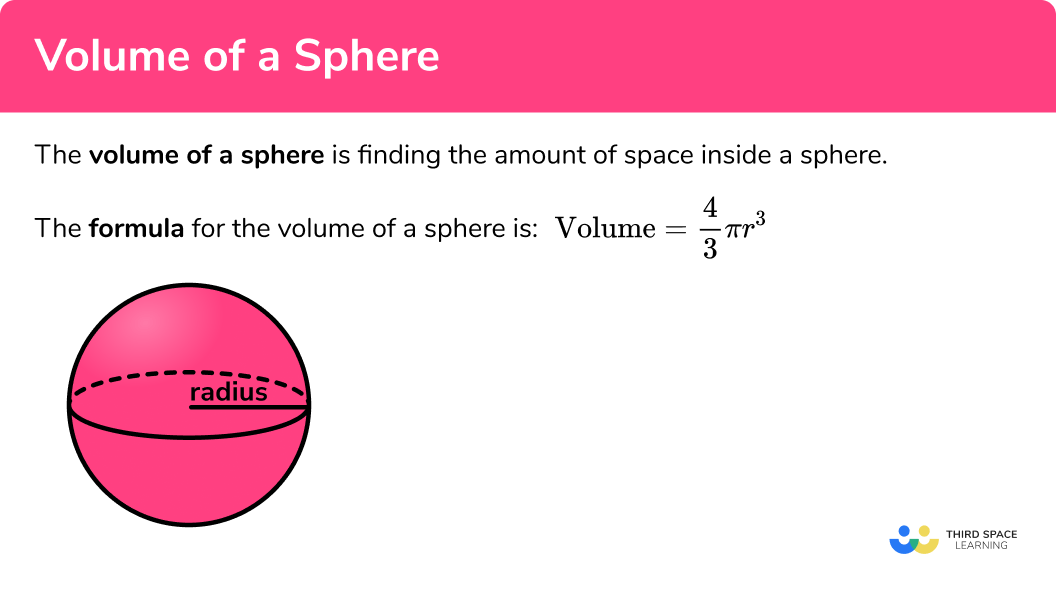## How to calculate the volume of a sphere

In order to calculate the volume of a sphere:

1. Write down the formula for the volume of a sphere.
2. Substitute given values into the formula.
3. Complete the calculation.
4. Write the answer, including the units.

### Explain how to calculate the volume of a sphere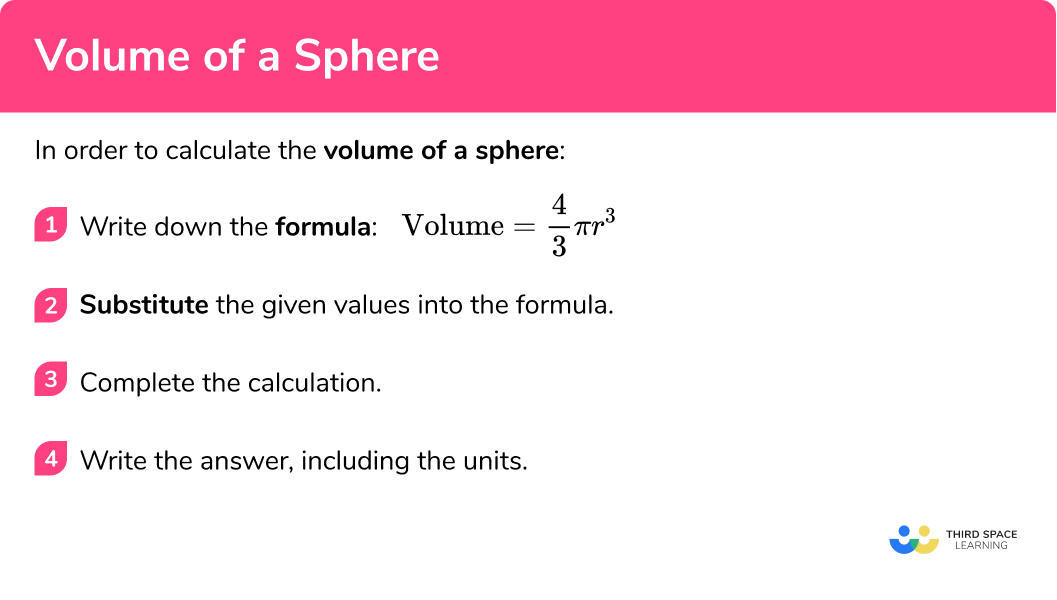### Related lessons on sphere

Volume of a sphere is part of our series of lessons to support revision on sphere. You may find it helpful to start with the main sphere lesson for a summary of what to expect, or use the step by step guides below for further detail on individual topics. Other lessons in this series include:

## Volume of a sphere examples

### Example 1: volume of a sphere given the radius

Calculate the volume of a sphere with radius 6 \; cm . Write your answer to 1 decimal place.

1. Write down the formula for the volume of a sphere.

The formula for the volume of a sphere is V=\frac{4}{3} \pi r^3 .

2Substitute the given values into the formula.

We need to substitute the value of the radius r=6 into the formula.

V=\frac{4}{3} \times \pi \times 6^3

3Complete the calculation.

Use a calculator to work out the volume.

V=288 \pi =904.7786842...

4Write the answer, including the units.

Here we are asked to give the answer to 1 decimal place.

The volume of the sphere is 904.8 \; cm^3 \; (1dp) .

Find the volume of a sphere with radius 7.2 \; cm . Write your answer to 2 decimal places.

V=\frac{4}{3} \pi r^3

Substituting the value r=7.2 \; cm into the formula for the volume of a sphere, we have

V=\frac{4}{3} \times \pi \times 7.2^3 .

Use a calculator to work out the volume.

V=1563.457566...

Here we are asked to write the answer to 2 decimal places.

V= 1563.457566… = 1563.46 \; cm^3 \; (2dp)

The volume of the sphere is 1563.46 \; cm^3 \; (2dp) .

### Example 3: volume of a sphere given the diameter – in terms of pi

Calculate the volume of a sphere with a diameter of 6m. Write your answer in terms of \pi .

V=\frac{4}{3} \pi r^3

The radius is half the length of the diameter and so

r=6\div{2}=3\text{m}.

We need to substitute the value of the radius into the formula.

V=\frac{4}{3} \times \pi \times 3^3

V=36\pi

Here we are asked to write the answer in terms of \pi .

V= 36\pi \text{ m}^3

The volume of the sphere is 36\pi \text{ m}^3 .

### Example 4: volume of a hemisphere

Find the volume of a hemisphere with radius 8 \; cm . Write your answer to 1 decimal places.

A hemisphere has half the volume of a sphere, so we need to divide the volume of a sphere by 2 ,

V=\frac{4}{3} \pi r^3 \div{2} .

Given that r=8 , we have

V=\frac{4}{3} \times \pi \times 8^3\div{2} .

Use a calculator to work out the volume.

V=\frac{1024}{3}\pi

Here we are asked to write the answer to 1 decimal places.

V=\frac{1024}{3}\pi = 1072.330292… = 1072.3 \text{ cm}^3 \ \text{(1dp)}

The volume of the hemisphere is 1072.3 \; cm^3 .

### Example 5: volume of a hemisphere

Find the volume of a hemisphere with radius 12.5 \; cm . Give your answer to 2 decimal places

Remember we have a hemisphere so we need to halve the volume of the equivalent sphere.

V=\frac{4}{3} \pi r^3 \div{2}

We need to substitute the value of the radius into the formula.

V=\frac{4}{3} \times \pi \times 12.5^3\div{2}

Use a calculator to work out the volume.

V=4090.615434...

Here we are asked to give the answer to 2 decimal places.

V=4090.615434...=4090.62 \; cm^3 \; (2dp)

The volume of the hemisphere is 4090.62 \; cm^3 \; (2dp) .

### Calculating the radius given the volume

In order to calculate the radius of a sphere given the volume, we need to rearrange the formula for the volume of a sphere (V=\frac{4}{3}\pi r^3) to make r (the radius) the subject of the formula.

Now we have the formula for determining the radius of a sphere given the volume to be,

r=\sqrt{\frac{3V}{4\pi}} .

## How to calculate the radius of a sphere given the volume

In order to calculate the radius of the sphere given the volume:

1. Write down the formula for the radius of a sphere, in terms of the volume.
2. Substitute the given values into the formula.
3. Complete the calculation.
4. Write the answer, including the units.

## Calculating the radius of a sphere examples

### Example 6: calculating the radius given the volume

The volume of a sphere is 3000 \; cm^3 . Calculate the radius of the sphere, correct to 2 decimal places.

r=\sqrt{\frac{3V}{4\pi}}

We are given the volume, so we can substitute this into the formula to calculate the radius,

r=\sqrt{\frac{3\times{3000}}{4\pi}} .

\begin{aligned} r&=\sqrt{\frac{9000}{4\pi}} \\\\ &=\sqrt{716.1972439...} \\\\ &=8.947002289... \end{aligned}

Here we are asked to give the answer to 2 decimal places.

r=8.947002289...=8.95 \; cm \; (2dp)

The radius of the sphere is 8.95 \; cm \; (2dp) .

### Example 7: calculating the radius given the volume

Calculate the radius of a sphere with the volume 8460 \; m^3 . Write your answer to the nearest centimetre.

Here we need to use

r=\sqrt{\frac{3V}{4\pi}} .

Substituting the value for the volume, we have

r=\sqrt{\frac{3\times{8460}}{4\pi}} .

\begin{aligned} r&=\sqrt{\frac{25380}{4\pi}} \\\\ &=\sqrt{2019.676228...} \\\\ &=12.64039323... \end{aligned}

Here we are asked to give the answer to the nearest centimetre.

r=12.64039323...=12.64 \; m=1264 \; cm

The radius of the sphere is 1264 \; cm .

### Common misconceptions

• Using the correct formula

There are several formulas that can be used, so we need to match the correct formula to the correct context.

• Rounding

It is important to not round the answer until the end of the calculation. This will mean your final answer is accurate. It is useful to keep your answer in terms of \pi until you round the answer at the very end of the question.

• Make sure you have the correct units

For area we use square units such as cm^2 .

For volume we use cube units such as cm^3 .

• Using the radius or the diameter

It is a common error to mix up radius and diameter. Remember the radius is half of the diameter.

### Practice volume of a sphere questions

1. Calculate the volume of the sphere. Write your answer to 1 decimal place.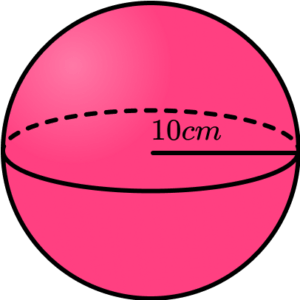4188.8 \; cm^3314.2 \; cm^31.3 \; cm^31256.6 \; cm^3We are finding the volume of a sphere so we substitute the value of r into the formula.

\begin{aligned} V&=\frac{4}{3} \pi r^3 \\\\ &= \frac{4}{3} \times \pi \times 10^3\\\\ &=\frac{4000}{3}\pi\\\\ &=4188.790205… \\\\ &=4188.8 \ cm^3 \ \text{(1dp)} \end{aligned}

2. Calculate the volume of the sphere with the diameter 13.6cm. Write your answer to 1 decimal place.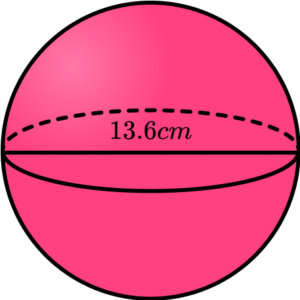10536.7 \; cm^32324.3 \; cm^31317.1 \; cm^3581.1 \; cm^3The radius is half the value of the diameter and so r=13.6 \div 2=6.8 \; cm.
Substituting r=6.8 into V=\frac{4}{3} \pi r^3 , we have
\begin{aligned} V&=\frac{4}{3} \pi r^3 \\\\ &= \frac{4}{3} \times \pi \times 6.8^3\\\\ &=1317.089682… \\\\ &=1317.1\text{cm}^3\text{ (1dp)} \end{aligned}

3. A sphere has a radius of 9m. Calculate the volume of the sphere in terms of \pi .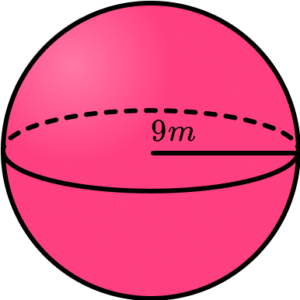243\pi \; m^3108\pi \; m^3972\pi \; m^3729\pi \; m^3Substituting r=9 into the formula for the volume of a sphere, we have

\begin{aligned} V&=\frac{4}{3} \pi r^3 \\\\ &= \frac{4}{3} \times \pi \times 9^3\\\\ &=972\pi \end{aligned}

4. Calculate the volume of the hemisphere. Write your answer to the nearest integer.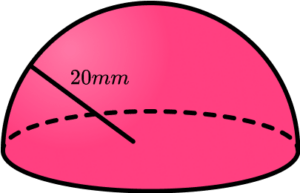33510 \; mm^316755 \; mm^31257 \; mm^33770 \; mm^3We are finding the volume of a hemisphere, so we need half of the volume of a sphere.

\begin{aligned} V&= (\frac{4}{3} \pi r^3) \div{2}\\\\ &= (\frac{4}{3} \times \pi \times 20^3) \div{2}\\\\ &=\frac{16 000}{3} \pi\\\\ &=16 755.16082… \\\\ &=16 755 \text{ mm}^3\text{ (0dp)} \end{aligned}

5. A hemisphere has a diameter of 24km. Calculate the volume of the hemisphere in terms of \pi .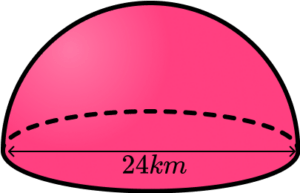2304\pi \; km^37240 \; km^31152\pi \; km^33620 \; km^3We are finding the volume of a hemisphere, so we need to calculate half of the volume of a sphere.

\begin{aligned} V&=\frac{4}{3} \pi r^3 \div{2}\\\\ &= \frac{4}{3} \times \pi \times 12^3 \div{2}\\\\ &=1152\pi \\\\ &=1152\pi \ km^3 \end{aligned}

6. A sphere has a volume of 1500 \; cm^3 . Calculate the radius of the sphere, correct to 1 decimal place.

7.1 \; cm18.9 \; cm37.8 \; cm11.3 \; cmUsing the formula for the radius in terms of the volume, we substitute the value of the volume and solve to find the radius.

\begin{aligned} r&=\sqrt{\frac{3V}{4\pi}} \\\\ &=\sqrt{\frac{3\times15000}{4\pi}} \\\\ &=\sqrt{358.098622…} \\\\ &=7.101240423… \\\\ &=7.1 \; cm \; (1dp) \end{aligned}

### Volume of a sphere GCSE questions

\text{Volume of a sphere}=\frac{4}{3} \pi r^3

1. Here is a sphere with a radius 10.4 \; cm.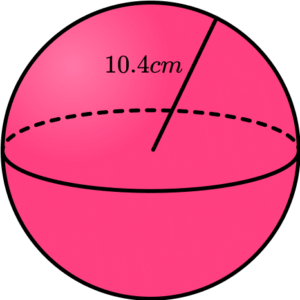Calculate the volume of the sphere. Write your answer to 1 decimal place.

(2 marks)

V=\frac{4}{3} \times \pi \times 10.4^3

(1)

4711.8 \; cm^3

(1)

2. (a) A model globe has a diameter of 30 \; cm. Calculate the volume of the globe in terms of \pi .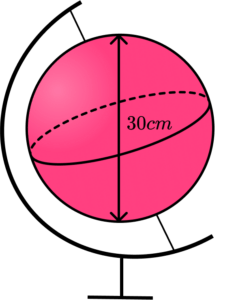(b) The mass of the model globe is 1.2kg, excluding the frame. Calculate the density in g/cm^3 of the model, to 2 decimal places.

(4 marks)

(a)

V=\frac{4}{3} \times \pi \times 15^3

(1)

4500\pi

(1)

(a)

\text{Density }=1200\div{4500\pi}

(1)

D=0.08\text{ g/cm}^3

(1)

3. A ceramic bowl is in the shape of a hemisphere. The bowl has a radius 25 \; cm.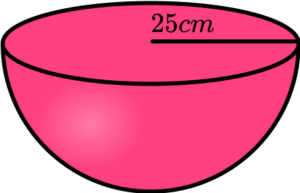Water fills the bowl at a rate of 500 \; cm^3 per minute. How long does it take to fill the bowl? Write your answer to the nearest second.

(3 marks)

\frac{4}{3} \times \pi \times 25^3 \div{2}= \frac{31 250}{3} \pi = 32724.92347…

(1)

32 724.923… \div 2000=16.36246174… or \frac{125}{24}\pi

(1)

16.36246174… minutes = 16 minutes 22 seconds

(1)

## Learning checklist

You have now learned how to:

• Calculate the volume of a sphere
• Calculate the volume of a hemisphere
• Solve problems involving the volume of a sphere

## Still stuck?

Prepare your KS4 students for maths GCSEs success with Third Space Learning. Weekly online one to one GCSE maths revision lessons delivered by expert maths tutors.

Find out more about our GCSE maths tuition programme.